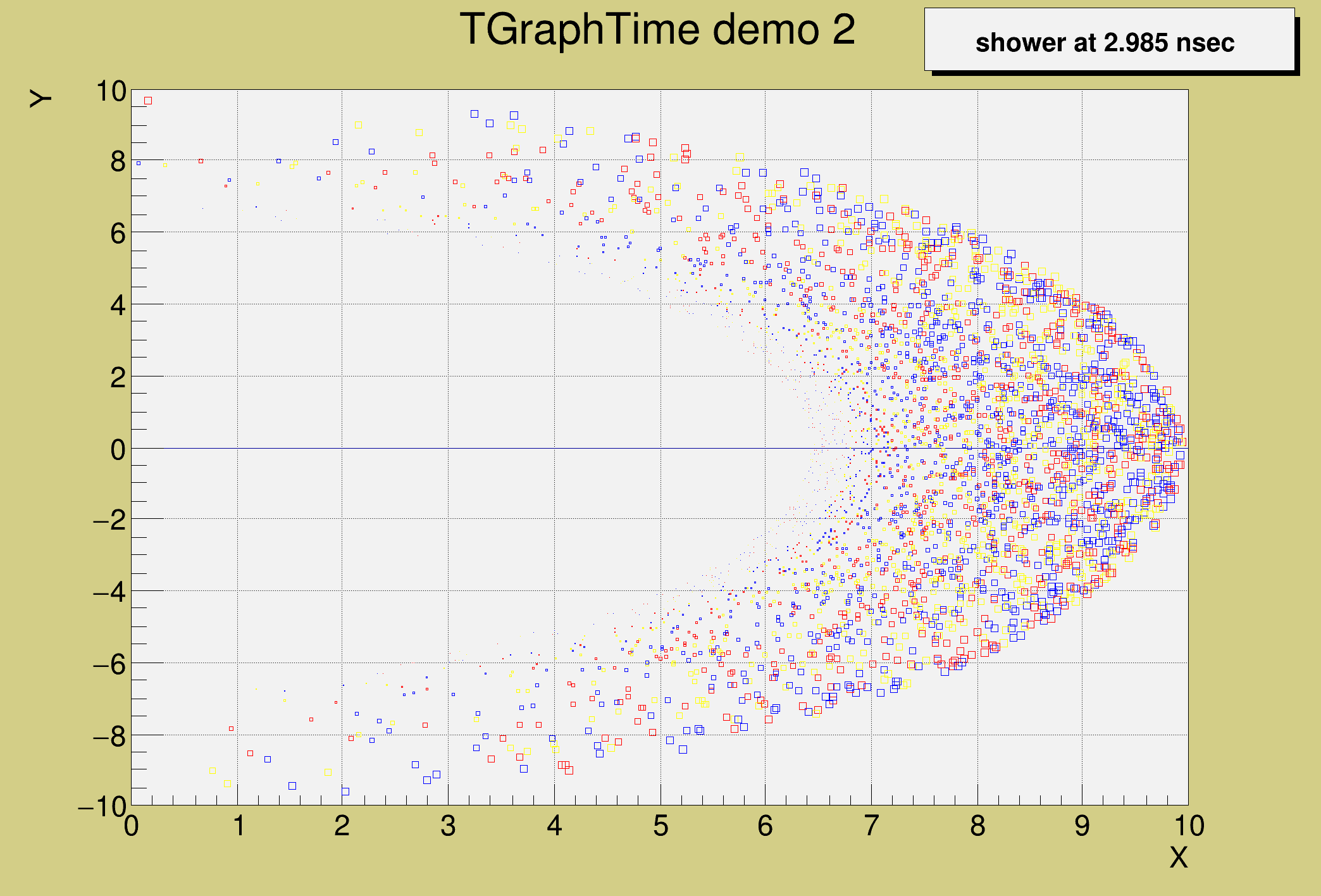ROOT   Reference Guidegtime2.C File Reference

## Detailed DescriptionExample of TGraphTime showing how the class could be used to visualize a set of particles with their time stamp in a MonteCarlo program.#include "TRandom3.h"
#include "TMath.h"
#include "TMarker.h"
#include "TPaveLabel.h"
#include "TArrow.h"
#include "TGraphTime.h"
void gtime2(Int_t nsteps = 200, Int_t np=5000) {
if (np > 5000) np = 5000;
Int_t color;
Double_t cosphi, sinphi, speed;
Double_t xmin = 0, xmax = 10, ymin = -10, ymax = 10;
g->SetTitle("TGraphTime demo 2;X;Y");
Int_t i,s;
Double_t phi,fact = xmax/Double_t(nsteps);
for (i=0;i<np;i++) { //calculate some object parameters
speed[i] = r.Uniform(0.5,1);
phi = r.Gaus(0,TMath::Pi()/6.);
cosphi[i] = fact*speed[i]*TMath::Cos(phi);
sinphi[i] = fact*speed[i]*TMath::Sin(phi);
Double_t rc = r.Rndm();
color[i] = kRed;
if (rc > 0.3) color[i] = kBlue;
if (rc > 0.7) color[i] = kYellow;
}
for (s=0;s<nsteps;s++) { //fill the TGraphTime step by step
for (i=0;i<np;i++) {
Double_t xx = s*cosphi[i];
if (xx < xmin) continue;
Double_t yy = s*sinphi[i];
TMarker *m = new TMarker(xx,yy,25);
m->SetMarkerColor(color[i]);
m->SetMarkerSize(1.5 -s/(speed[i]*nsteps));
}
}
g->Draw();
}
ROOT::R::TRInterface & r
Definition: Object.C:4
#define g(i)
Definition: RSha256.hxx:105
int Int_t
Definition: RtypesCore.h:45
double Double_t
Definition: RtypesCore.h:59
@ kRed
Definition: Rtypes.h:66
@ kBlue
Definition: Rtypes.h:66
@ kYellow
Definition: Rtypes.h:66
float xmin
Definition: THbookFile.cxx:95
float ymin
Definition: THbookFile.cxx:95
float xmax
Definition: THbookFile.cxx:95
float ymax
Definition: THbookFile.cxx:95
char * Form(const char *fmt,...)
TGraphTime is used to draw a set of objects evolving with nsteps in time between tmin and tmax.
Definition: TGraphTime.h:29
Manages Markers.
Definition: TMarker.h:22
A Pave (see TPave) with a text centered in the Pave.
Definition: TPaveLabel.h:20
Random number generator class based on M.
Definition: TRandom3.h:27
static constexpr double s
Double_t Cos(Double_t)
Definition: TMath.h:643
constexpr Double_t Pi()
Definition: TMath.h:37
Double_t Sin(Double_t)
Definition: TMath.h:639
auto * m
Definition: textangle.C:8

Definition in file gtime2.C.